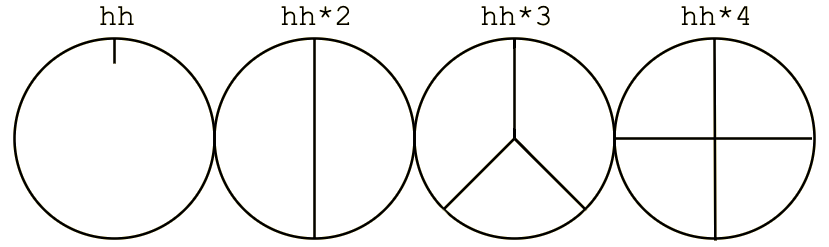# Cycles

Tidal Cycles is not using BPM (beats per minute) but a specific measurement called CPS: cycles per second. For Tidal, time is cyclical and not linear. It means that when a cycle ends, a new one will follow. Time is counted in smaller and smaller decrements of cycles per second (e.g. 1/3 of a cycle).This rather original way of dealing with time can be quite surprising for a musician, because both traditional european notation and modern sequencers are generally linear and deal with the beginning of time and the ending of time. Tidal can backtrack or fastforward in time because you can actually predict what will happen in `x` cycles or what happened `x` cycles ago (well, kinda..).

## Dividing the cycle​

Don't focus on the syntax so far! Enter the following pattern in your text editor and evaluate it:

``d1 \$ s "bd hh bd hh"``

You just divided a cycle in four equal parts, one for each of the sounds you just triggered. However:

``d1 \$ s "bd hh hh"``

Now, the cycle is being divided in three equal parts, you might have noticed that it slowed down a little.

You can superpose patterns that will divide the `cycle` in different subdivisions. It means that Tidal is a rather good tool to explore polyrhythmy and rhythmic intricacies:

``d1 \$ s "bd hh hh"d2 \$ s "hh:2 ~ hh:3 cp"``

## Visualizing cycles​Tidal can help you to visualize the output of a given pattern textually or graphically. tidal-vis can go even further by turning textual patterns into their visual counterpart. Enter the following pattern:

``"1 2 3"``

You should see this result in the logs (the `ghci` window):

``(0>⅓)|"1"(⅓>⅔)|"2"(⅔>1)|"3"``

You can also use the `drawLine` function to visualize the output of a pattern in the log console.

``drawLine "a b*2 d"``

You might get something that looks like this:

``[11 cycles]|a-bbd-|a-bbd-|a-bbd-|a-bbd-|a-bbd-|a-bbd-|a-bbd-|a-bbd-|a-bbd-|a-bbd-|a-bbd-``

## Convert between BPM and CPS​

Sometimes, you will need to convert between BPMs and CPS (e.g. synchronization with another musician or machine). The `setcps` function is used to change the number of cycles per second. The default number of cycles per second is 0.5625.

These two values are equivalent:

• `setcps 0.5625`: Cycles per second, as a decimal.
• `setcps (135/60/4)`: Cycles per second, as a fraction.

Representing cycles per second using fractions has the advantage of being more human-readable and more closely aligned with how tempo is commonly represented in music as beats per minute (or bpm). Techno has a range of 120-140 bpm. House has a range of 115-130 bpm. And so on. If we wanted to set the tempo of our Tidal song to fast house, we would do the following:

``-- Set cps to be a fast house beatsetcps (130/60/4)``

Regarding the example above, the first part of the fraction `130/60`, says there will be 130 beats per minute. 130 is the number of beats and 60 is the length of the minute (60 seconds). The second part of the fraction `/4` says that for every cycle in tidal there will be 4 beats. You can adjust this value to change how quickly your cycles run.

## Pop-up window​

You can use SuperCollider GUI libraries to create a small window showing the current state of the Tidal Clock. `pulu` scripted the following solution:

``// start superdirt first(var clockMods, clockBeats, screenW, screenH, clockW, clockH, clockX, clockY, resizable, border;clockMods = [4,6];clockBeats = 4;screenW = 1440;screenH = 900;clockW = 120;clockH = 22;clockX = screenW - clockW;clockY = screenH - 1;resizable = false;border = false;~clockText = StaticText().string_("[clock]").font_(Font.defaultMonoFace).align_(\center).stringColor_(Color(1,1,1)).minHeight_(20);~updateClock = { |cycle|    var text, beat;    text = clockMods.collect { |m| "" ++ (cycle.floor.asInteger.mod(m) + 1) ++ "/" ++ m; }.join(" ");    beat = (cycle.mod(1)*clockBeats).round.asInteger + 1;    text = text ++ " " ++ clockBeats.collect { |i| if(i < beat, ".", " "); }.join;    ~clockText.string_(text);};~clockWindow = Window("clock", Rect(clockX, clockY, clockW, clockH), resizable, border).background_(Color(0.3,0.3,0.3)).layout_(    HLayout(        ~clockText    ).margins_(0!4));~clockWindow.alwaysOnTop_(true);~clockWindow.visible_(true);SynthDef(\tick, { |cycle|    SendReply.kr(Impulse.kr(0), "/tick", [cycle]);    FreeSelf.kr(Impulse.kr(0));}).add;OSCdef(\tick, { |msg|    var cycle;    #cycle = msg[3..];    Routine {        { ~updateClock.(cycle); }.defer;    }.play(SystemClock);}, "/tick");)``

After evaluating this script (in your `BootTidal.hs` or after booting SuperDirt), play the following pattern:

``p "tick" \$ "0*4" # s "tick"``